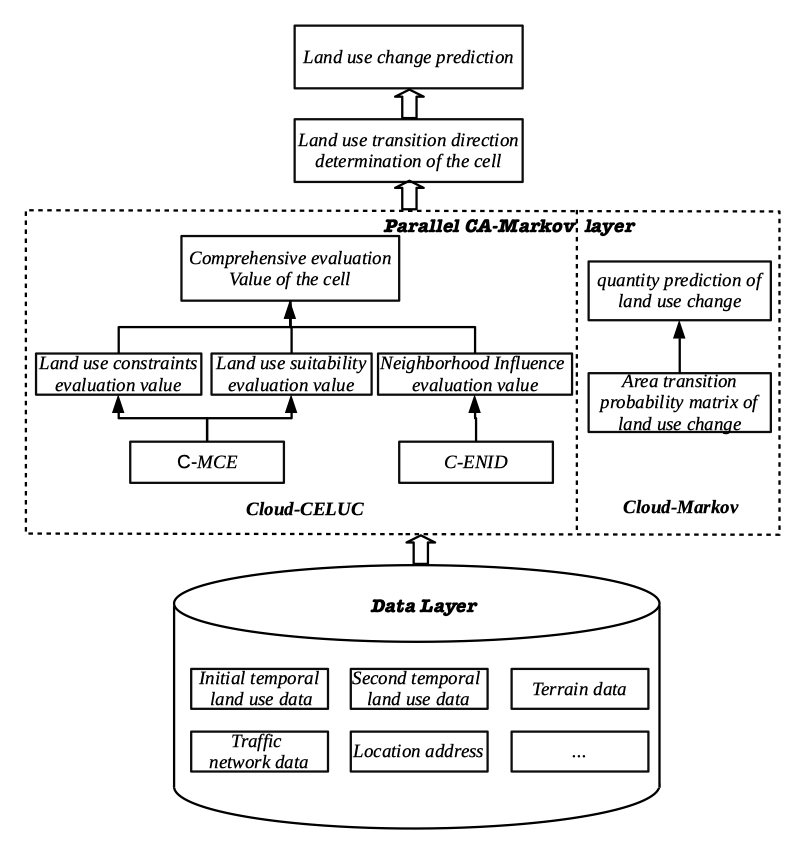# Land use and Land Cover modelling and prediction

CA Markov Model for Land use and Land Cover modelling and predictionThe Markov model is based on the theory of random processes. In this model, given the initial state
and state-transition probabilities, simulation results have nothing to do with the historical condition
before the current condition, which can be used to describe land-use changes from one period to
another. We can also use this as a basis to predict future changes. Change was found by creating a
land-use-change transition-probability matrix from periods
t to t + 1, which is the basis to predict
future land-use changes.

 S(t + 1) = Pij × S(t)

S(t + 1) denotes the state of the land-use system at times t + 1 and t, respectively. Pij is the
state-transition matrix.

CA has four basic components: Cell and cell space, cell state, neighborhood, and transition rules. The CA model can be expressed as follows

S(t + 1) = f(S(t), N)

In the formula, S is a state set of a finite and discrete state, t and t + 1 are different moments, N
is the neighborhood of the cell, and f is the cell-transition rule of the local space.
Usually, in order to make cellular automata better simulate a real environment, space constraint
variable
β needs to be introduced to express the topographic terrain, as well as the adaptive
constraints and restrictive constraints of the spatial-influence factors on the cells.

 S(t + 1) = f(S(t), N, β)

The separate Markov model lacks spatial knowledge and does not consider the spatial
distribution of geographic factors and land-use types, while the CA-Markov model adds spatial
features to the Markov model, uses a cellular-automata filter to create weight factors with spatial
character, and changes the state of the cells according to the state of adjacent cells and the transition rules.